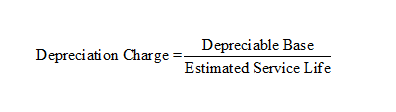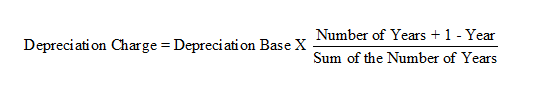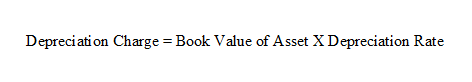# Depreciation, Amortization, Depletion, and Impairment

Depreciation, amortization, depletion, and impairment are ways of accounting the using up or decline in value of long lived assets.

• Depreciation is the process of allocating the cost of tangible assets to expense in a rational and systematic manner in the periods that the assets provide benefits.
• Amortization is the process of allocating the cost of intangible assets to expense in a rational and systematic manner in the periods that the assets provide benefits.
• Depletion is the process of recording the extraction of natural resources (wasting assets) to expense.
• Impairment is the process of recording the cost of an asset whose value lessoned to expense.

### Three things needed to compute depreciation

1. The depreciable base for the asset
2. The asset’s useful life
3. The depreciation method

Depreciable Base = Asset Cost – Salvage Value

### Depreciation Methods

1. Straight Line Method
2. Activity Method
3. Sum-of-the-Years'-digits Method
4. Declining Balance Method

### Straight Line Method

The straight-line method uniformly charges depreciation expense each of the asset’s service life### Activity Method

The activity method of deprecation is measure by a function of productivity. The depreciable base is multiplied by a ratio of the units of productivity divided by estimated total productivity. The units of productivity could be miles, produced units, hours.### Sum-of-the-Digits Method

The Sum-of-the-Digits method is an accelerated depreciation method that heavily weighs depreciation to the early part of the assets life.### Declining-Balance Method

The declining-balance method is an accelerated depreciation method applies the same ratio each period to the current value of the asset, ignoring salvage value. The percentage used is usual a multiple of straight-line depreciation rate.